output.to from Sideway
Mechanics: Statics

1

Draft for Information Only

# Content

```Second Moment of An Area of Geometric Shape  Moment of Inertia of Areas   Second Moment of Area of Rectangle    Second Moment about x' by Double Integration    Second Moment about y' by Single Integration    Second Moment about x by Parallel-Axis Theorem    Second Moment about y by Parallel-Axis Theorem    Polar Moment about C from Rectangular Moments of Inertia    Second Moment of Area of Circle    Second Moment about x'  by Double Integration    Second Moment about y'  by Double Integration    Polar Moment about C from Rectangular Moments of Inertia     Second Moment about A by Parallel-Axis Theorem   Second Moment of Area of Triangle    Second Moment about x' by Single Integration    Second Moment about x by Parallel-Axis Theorem```

# Second Moment of An Area of Geometric Shape

The second moment of an area of a geometric shape can be determined by integration or the parallel-axis theorem. Imply## Moment of Inertia of Areas

### Second Moment of Area of Rectangle

#### Second Moment about x' by Double Integration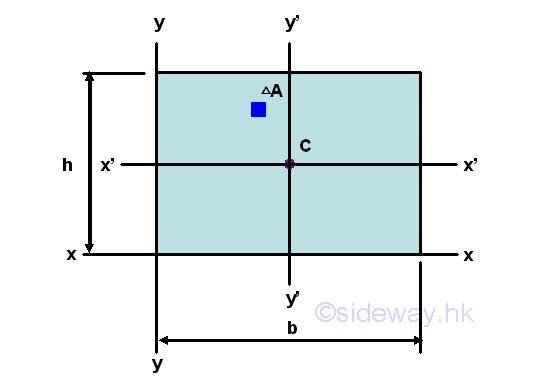The second moment of an area of a rectangle about the centroidal axis x' is#### Second Moment about y' by Single Integration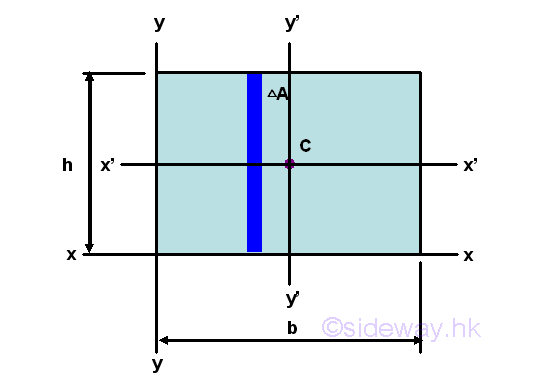The second moment of an area of a rectangle about the centroidal axis y' is#### Second Moment about x by Parallel-Axis Theorem

The second moment of an area of a rectangle about the axis x is#### Second Moment about y by Parallel-Axis Theorem

The second moment of an area of a rectangle about the axis y is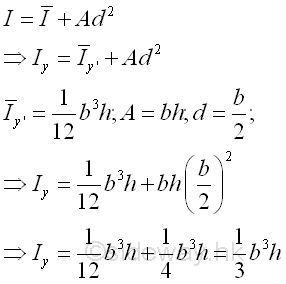#### Polar Moment about C from Rectangular Moments of Inertia

The polar moment of an area of a rectangle about the centroid C is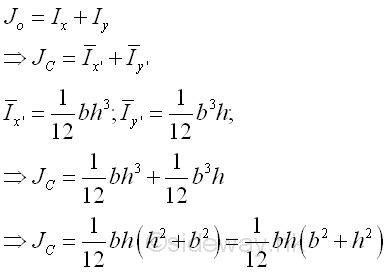### Second Moment of Area of Circle

#### Second Moment about x'  by Double IntegrationThe second moment of an area of a circle about the centroidal axis x' is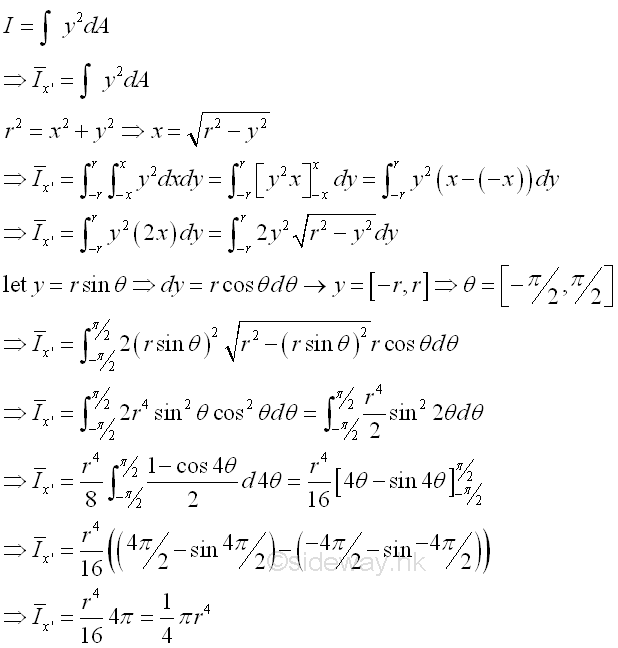#### Second Moment about y'  by Double Integration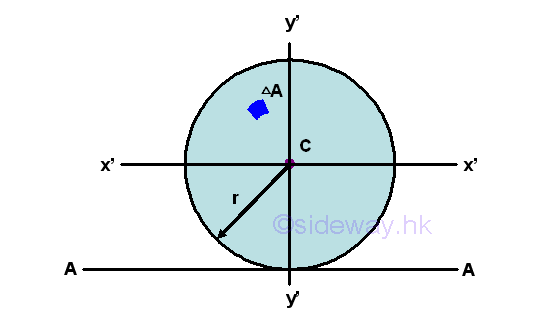The second moment of an area of a circle about the centroidal axis y' is#### Polar Moment about C from Rectangular Moments of Inertia

The polar moment of an area of a circle about the centroid C is#### Second Moment about A by Parallel-Axis Theorem

The second moment of an area of a rectangle about axis A is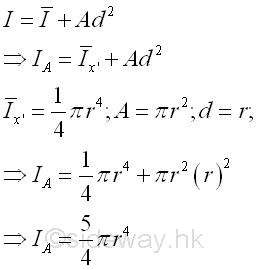### Second Moment of Area of Triangle

#### Second Moment about x' by Single Integration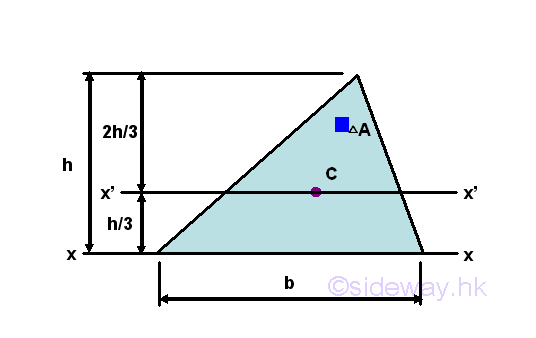The second moment of an area of a triangle about the centroidal axis x' is#### Second Moment about x by Parallel-Axis Theorem

The second moment of an area of a triangle about the axis x is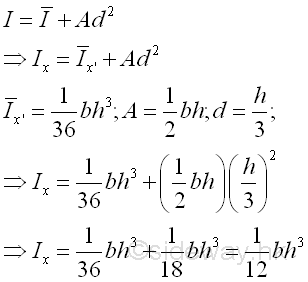©sidewayReferences

1. I.C. Jong; B.G. rogers, 1991, Engineering Mechanics: Statics and Dynamics, Saunders College Publishing, United States of America
2. F.P. Beer; E.R. Johnston,Jr.; E.R. Eisenberg, 2004, Vector Mechanics for Engineers: Statics, McGraw-Hill Companies, Inc., New YorkID: 121000007 Last Updated: 2012/10/16 Revision: 0 Ref:Home (5)

Business

Management

HBR (3)

Information

Recreation

Hobbies (7)

Culture

Chinese (1097)

English (336)

Reference (66)

Computer

Hardware (149)

Software

Application (187)

Digitization (24)

Numeric (19)

Programming

Web (648)CSS (SC)

ASP.NET (SC)

HTML

Knowledge Base

Common Color (SC)

Html 401 Special (SC)

OS (389)

MS Windows

Windows10 (SC)

.NET Framework (SC)

DeskTop (7)

Knowledge

Mathematics

Formulas (8)

Number Theory (206)

Algebra (20)

Trigonometry (18)

Geometry (18)

Calculus (67)

Complex Analysis (21)

Engineering

Tables (8)

Mechanical

Mechanics (1)

Rigid Bodies

Statics (92)

Dynamics (37)

Fluid (5)

Control

Acoustics (19)

Biology (1)

Geography (1)

Copyright © 2000-2019 Sideway . All rights reserved Disclaimers last modified on 10 Feb 2019